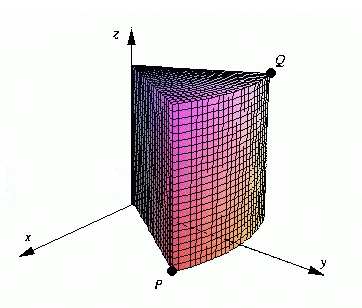# Triple integral with an image given

## Homework Statement

Integrate the function f(xyz)=−4x+6y over the solid given by the figure below, if P = (5,1,0) and Q = (-5,1,5).where P=(5,1,0) and Q=(-5,1,5)

r²=x²+y²
tan theta=y/x
z=z
y=rsintheta
x=rcostheta

## The Attempt at a Solution

So I treated this as a cylindrical coordinates and first changed f(x,y,z)=6y-4x into f(r, theta, z)=6rsin(th)-4rcos(th)

Then I got the limits by finding r which is sqrt(26). 0<=r<=sqrt(26)
Next, I loked for theta so I that's -arctan(5)<=theta<=arctan(5)
Finally z=z so z goes from 0 to 5 as shown in the picture. 0<=z<=5

I integrated all this and got 1733.3333333333, which was wrong. Can someone tell me what I'm doing here?

Mark44
Mentor

## Homework Statement

Integrate the function f(xyz)=−4x+6y over the solid given by the figure below, if P = (5,1,0) and Q = (-5,1,5).where P=(5,1,0) and Q=(-5,1,5)

r²=x²+y²
tan theta=y/x
z=z
y=rsintheta
x=rcostheta

## The Attempt at a Solution

So I treated this as a cylindrical coordinates and first changed f(x,y,z)=6y-4x into f(r, theta, z)=6rsin(th)-4rcos(th)

Then I got the limits by finding r which is sqrt(26). 0<=r<=sqrt(26)
Next, I loked for theta so I that's -arctan(5)<=theta<=arctan(5)
Finally z=z so z goes from 0 to 5 as shown in the picture. 0<=z<=5

I integrated all this and got 1733.3333333333, which was wrong. Can someone tell me what I'm doing here?

Your range for theta is wrong. It is -1/5 <= theta <= 1/5.

ok i changed the theta and got 346.. but the answer is still wrong =[

Mark44
Mentor
ok i changed the theta and got 346.. but the answer is still wrong =[
Show what you did and we'll see if we can find what's wrong.